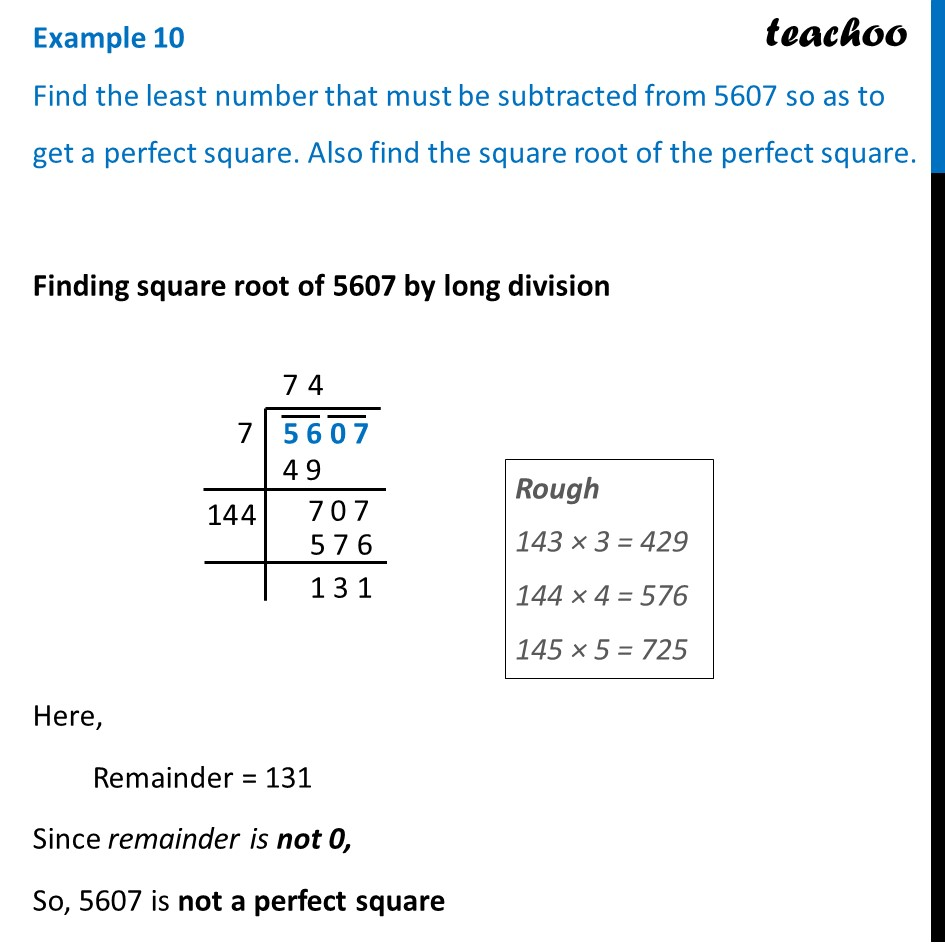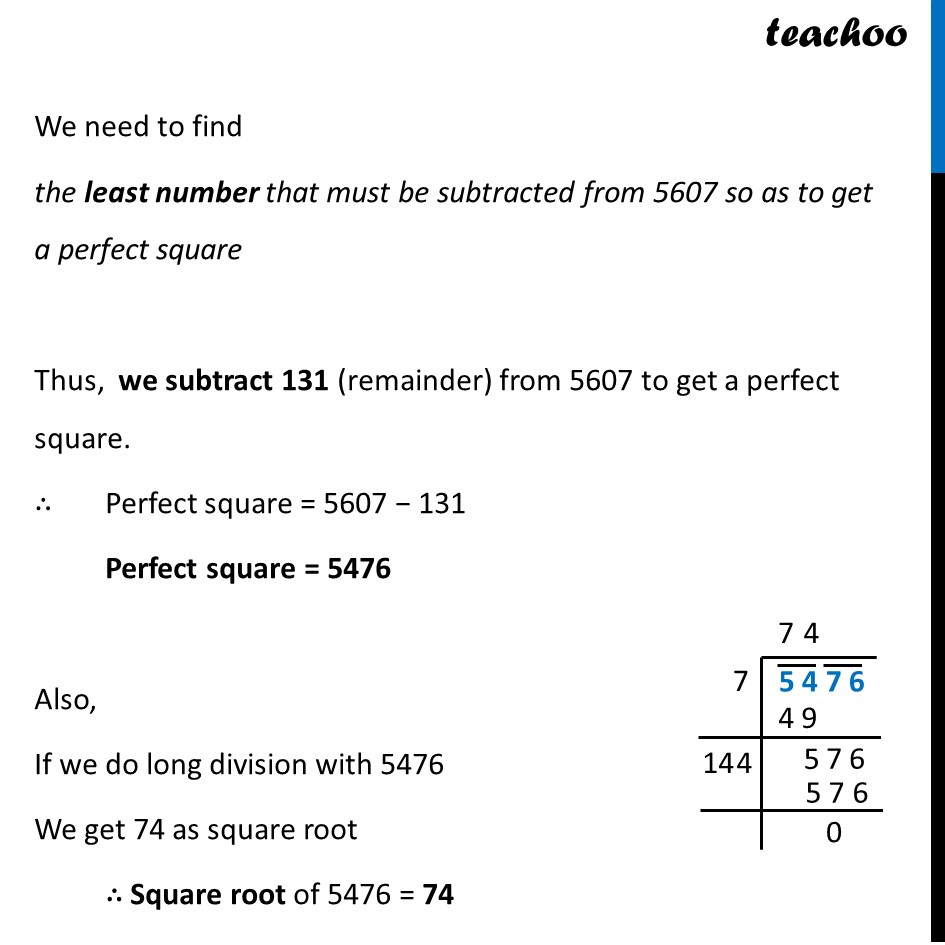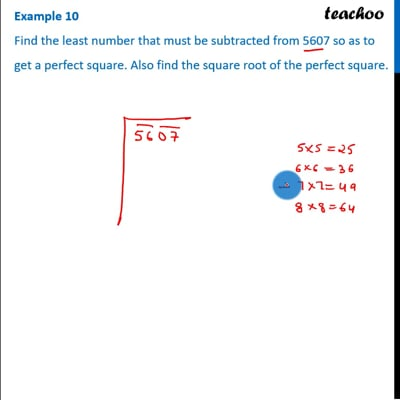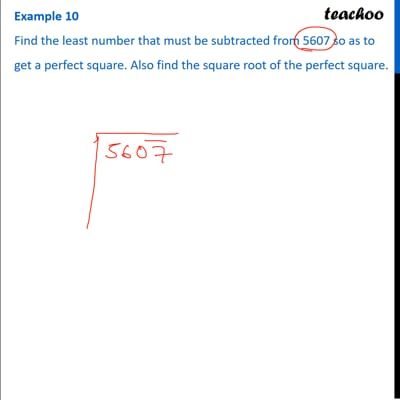Examples

Chapter 5 Class 8 Squares and Square Roots
Serial order wiseThis video is only available for Teachoo black usersThis video is only available for Teachoo black usersThis video is only available for Teachoo black users

Learn in your speed, with individual attention - Teachoo Maths 1-on-1 Class

### Transcript

Example 10 Find the least number that must be subtracted from 5607 so as to get a perfect square. Also find the square root of the perfect square. Finding square root of 5607 by long division Finding square root of 5607 by long division Here, Remainder = 131 Since remainder is not 0, So, 5607 is not a perfect square We need to find the least number that must be subtracted from 5607 so as to get a perfect square Thus, we subtract 131 (remainder) from 5607 to get a perfect square. ∴ Perfect square = 5607 − 131 Perfect square = 5476 Also, If we do long division with 5476 We get 74 as square root ∴ Square root of 5476 = 74